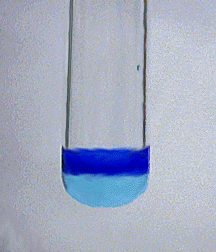# Characteristic Reactions of Copper Ions (Cu²⁺)

$$\newcommand{\vecs}{\overset { \rightharpoonup} {\mathbf{#1}} }$$ $$\newcommand{\vecd}{\overset{-\!-\!\rightharpoonup}{\vphantom{a}\smash {#1}}}$$$$\newcommand{\id}{\mathrm{id}}$$ $$\newcommand{\Span}{\mathrm{span}}$$ $$\newcommand{\kernel}{\mathrm{null}\,}$$ $$\newcommand{\range}{\mathrm{range}\,}$$ $$\newcommand{\RealPart}{\mathrm{Re}}$$ $$\newcommand{\ImaginaryPart}{\mathrm{Im}}$$ $$\newcommand{\Argument}{\mathrm{Arg}}$$ $$\newcommand{\norm}{\| #1 \|}$$ $$\newcommand{\inner}{\langle #1, #2 \rangle}$$ $$\newcommand{\Span}{\mathrm{span}}$$ $$\newcommand{\id}{\mathrm{id}}$$ $$\newcommand{\Span}{\mathrm{span}}$$ $$\newcommand{\kernel}{\mathrm{null}\,}$$ $$\newcommand{\range}{\mathrm{range}\,}$$ $$\newcommand{\RealPart}{\mathrm{Re}}$$ $$\newcommand{\ImaginaryPart}{\mathrm{Im}}$$ $$\newcommand{\Argument}{\mathrm{Arg}}$$ $$\newcommand{\norm}{\| #1 \|}$$ $$\newcommand{\inner}{\langle #1, #2 \rangle}$$ $$\newcommand{\Span}{\mathrm{span}}$$$$\newcommand{\AA}{\unicode[.8,0]{x212B}}$$

• Most common oxidation states: +1, +2
• M.P. 1083º
• B.P. 2582º
• Density 8.92 g/cm3
• Characteristics: Copper is a reddish-yellow, rather inactive metal. Dissolves readily in $$\ce{HNO3}$$ and in hot, concentrated $$\ce{H2SO4}$$.

## Characteristic reactions of Cu²⁺

The +2 oxidation state is more common than the +1. Copper(II) is commonly found as the blue hydrated ion, $$\ce{[Cu(H2O)4]^{2+}}$$.

### Aqueous Ammonia

Copper(II) ion reacts with stoichiometric quantities of aqueous ammonia to precipitate light blue Cu(OH)2. Some basic salts may also form.

$\ce{Cu2+(aq) + 2NH3(aq) + 3H2O(l) <=> Cu(OH)2(s) + 2NH4+(aq)}$The precipitate dissolves in excess ammonia to form a dark blue complex ion:

$\ce{Cu(OH)2(s) + 4NH3(aq) <=> [Cu(NH3)4]2+(aq) + 2OH-(aq) }$### Sodium Hydroxide

Sodium hydroxide precipitates copper(II) hydroxide:

$\ce{Cu2+(aq) + 2OH-(aq) <=> Cu(OH)2(s)}$The precipitate does not dissolve in excess sodium hydroxide unless the NaOH solution is very concentrated. However, the precipitate will dissolve upon addition of concentrated ammonia solution.

### Potassium Ferrocyanide

Potassium ferrocyanide precipitates red-brown copper(II) ferrocyanide from Cu2+ solutions:

$\ce{2Cu2+(aq) + [Fe(CN)6]4-(aq) <=> Cu2[Fe(CN)6](s)}$This test is very sensitive. The precipitate is soluble in aqueous ammonia.

Note: Many metal ions form ferrocyanide precipitates, so potassium ferrocyanide is not a good reagent for separating metal ions. It is used more commonly as a confirmatory test.

### No Reaction

$$\ce{Cl^{-}}$$, $$\ce{SO4^{2-}}$$

This page titled Characteristic Reactions of Copper Ions (Cu²⁺) is shared under a CC BY-NC-SA 4.0 license and was authored, remixed, and/or curated by James P. Birk.